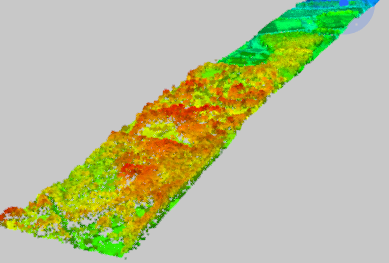# Point Cloud

A point cloud geometry is a (potentially large) collection of points. Point clouds are useful for storing large amounts of data, often gathered from LIDAR applications.Associated with each point are properties called components, which contain a value that describes the point. Components are similar to attributes, but at the point level instead of at the feature level. Note that all points in a point cloud have the same schema (that is, the same set of components), but each point may have a different value for each component.

All components have a name and type. Possible component types are Real64, Real32, UInt64, UInt32, UInt16, UInt8, Int64, Int32, Int16, Int8, and String. Numeric components additionally may have a scale and offset factor; in such cases, the applied value of the component is `value x scale + offset`. String components additionally may have an encoding.

While components may have any name, there are a few common components that exist in several formats. These components are described in the following table.

Note  This list is not exhaustive. A point cloud may have any number of components not listed here.
Component Name Description
x The x component of the geometry.
y The y component of the geometry.
z The z component of the geometry.
intensity The magnitude of the intensity of the pulse return.
color_red The red image channel value at the point.
color_green The green image channel value at the point.
color_blue The blue image channel value at the point.
classification The class of the point. Categorizes a point into fields, such as ground, building, water, and others. Values correspond to the ASPRS LAS specification.
return The pulse return number for a given output pulse.
number_of_returns The total number of detected returns from a single pulse.
gps_time The number of seconds since the beginning of the week.
gps_week The week number, counting from January 6th, 1980.
angle The angle of the pulse that the point was scanned at.
flight_line The flight line number the point was detected in.
flight_line_edge Whether this point lies on the edge of the scan, along the flight line.
scan_direction The direction in which a scanning mirror was directed when the point was detected.
point_source_id A value that indicates the source of the file, such as a file number.
posix_time Used to express the time, as the number of seconds elapsed since UTC January 1st, 1970.
user_data Data to be used at the user’s discretion.
normal_x The x component of the vertex normals.
normal_y The y component of the vertex normals.
normal_z The z component of the vertex normals.

classification_flags

Indicates special characteristics associated with each point. Values correspond to the ASPRS LAS specification.

Note that there are no required components. For example, point clouds typically have all of the x/y/z components, but a point cloud may not have z (making it 2D), or may not have any of x/y/z (making it non-spatial).

Point clouds may also store a 4x4 transformation matrix, which is applied to the x/y/z components.

### Point Cloud Attributes

• fme_geometry = fme_polygon
• fme_type = fme_point_cloud